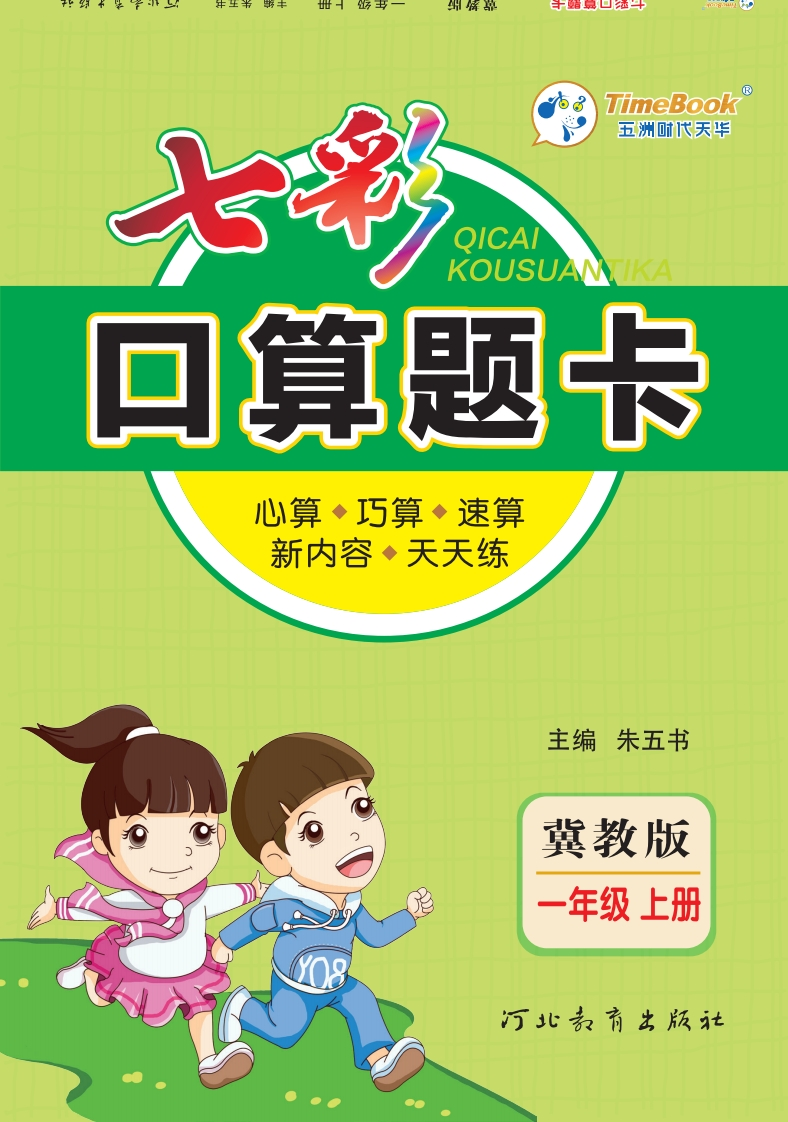##408

0

0
PDF## 七彩口算冀教版1年级上册-PDF电子文档总页数 178 页

TimeBookKOUSUAN可算题卡心算◆巧算◆速算新内容·天天练主编朱五书冀教版一年级上册1+1=22+1=31+2=33+1=42+2=41+3=44+1=53+2=52+3=51+4=55+1=64十2=63+3=62+4=61+5=66+1=75+2=74+3=73+4=72+5=71+6=77+1=86+2=85+3=84+4=83+5=82+6=81+7=88+1=97+2=96+3=95+4=94+5=93+6=92+7=91+8=99+1=108+2=107+3=106+4=105+5=104+6=103+7=102+8=101+9=102-1=13-1=23-2=14-1=34-2=24-3=15-1=45-2=35-3=25-4=16-1=56-2=46-3=36-4=26-5=17-1=67-2=57-3=47-4=37-5=27-6=18-1=78-2=68-3=58-4=48-5=38-6=28-7=19-1=89-2=79-3=69-4=59-5=49-6=39-7=29-8=110-1=910-2=810-3=710-4=610-5=510-6=410-7=310-8=210-9=1《七彩口算题卡》系列丛书多年来得到了广大读者的支持与厚爱，欢迎老师、家长和学生通过电话、写信等方式和我们联系，提出宝贵建议。我们将不断开拓创新，为广大读者服务。读者服务热线：0311-66538208转708地址：河北省石家庄市裕华区建设南大街235号金如意商务大厦16层邮编：0500241、本文档共计 178 页，下载后文档不带水印，支持完整阅读内容或进行编辑。
2、当您付费下载文档后，您只拥有了使用权限，并不意味着购买了版权，文档只能用于自身使用，不得用于其他商业用途（如 [转卖]进行直接盈利或[编辑后售卖]进行间接盈利）。
3、本站所有内容均由合作方或网友上传，本站不对文档的完整性、权威性及其观点立场正确性做任何保证或承诺！文档内容仅供研究参考，付费前请自行鉴别。
4、如文档内容存在违规，或者侵犯商业秘密、侵犯著作权等，请点击“违规举报”。